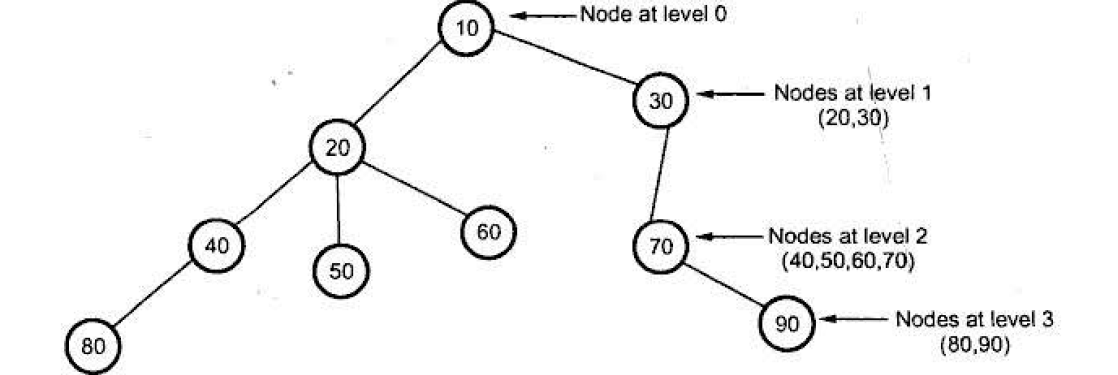# Data Structures

## Trees

A tree is a finite set of one or more nodes such that -
i)  Root node: It is a specially designated node.
ii) There are remaining n nodes which can be partitioned into disjoint sets A1, A2, A3 ... An where  A2, A3... An are called subtrees of the root.#### Root

Root is a unique node in the tree to which further subtrees arc attached. For above given tree, node A1 is a root node.

Parent node

The node haviog further sub-branches is called parent node. In Fig. 2 , 20  is parent node of 40, 50 and 60.Fig 2

Child Nodes

The child nodes in above given tree are marked as shown below.Fig 3

Leaves

These are the terminal nodes of the tree.

Degree of the node

The total number of sub-trees attached to that node is called the degree of a node

For exampleFig 4

Degree of tree

The maximum degree in the tree is degree of tree.Fig 5

Level of the tree
The root node is always considered at level zero.
The adjacent nodes to root are supposed to be at level 1 and so on.Fig 6

Height of the tree
The maximum level is the height of the tree. In fig 6 given above   the height of tree is 3. Sometimes height of the tree is also called depth of tree.

Predecessor
While displaying the tree, if some particular node occurs previous to some other node then that node is called predecessor of the other node.
For example : While displaying the tree in Fig 6  if we read node 20 first and then if we read node 40, Ihen 20 is a predecessor of 40.

Successor
Successor is a node which occurs next to some node.
For example: While displaying tree in Fig. 6  if we read node 60 after reading node 20 then 60 is called successor of 20.